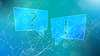# TechTopics No. 10

Heat generation estimation for Series 81000™ controllers

We are often asked to provide estimated heat generation data for our equipment. This paper provides information that allows calculation of approximate heat generated by the Series 81000™ motor controller equipment under assumed loading conditions.

The heat generation data given in the table for motor controllers is on the basis of full load operation at 1.0 service factor, and assumes that each controller includes a 0.75 kVA control power transformer that is only lightly loaded when the motor is running. For the main bus, the data assumes operation at full rated continuous current.

To estimate the heat generated under actual loading conditions, determine the component heat generation for each of the components indicated in the table. For simplicity, assume that main bus loading in each vertical section is equal to the total estimated load of the controller lineup, and adjust the heat generated for each vertical section by the square of the percentage of total estimated load to rated main bus current. Space heater load should be assumed to be continuous.

Table 1: Approximate full-load heat generation (in watts (W)) for Series 81000™ motor controllers
Motor controller fuse size
Maximum FLA
FVNR
RVAT

2R

3R

4R

6R

9R

12R

18R

24R

36R

26 A

46 A

64 A

88 A

123 A

183 A

272 A

360 A

420 A

440 W

545 W

645 W

740 W

775 W

910 W

1,290 W

1,570 W

2,090 W

710 W

850 W

975 W

1,110 W

1,215 W

1,485 W

1,930 W

2,530 W

3,290 W

Vertical section with main bus

600 A rated current

1,200 A rated current

2,000 A rated current

60 W

185 W

265 W

Space heaters, per vertical section

125 W
Voltage transformer trunnion

50 W
Conversion factor: Watts x 3.415179 = BTU/hour